## GOING UP ? [Day 2] - VISUAL MATH TALK

Represent, describe and solve contextual problems involving addition and subtraction of integers.

## Intentionality & Unit Overview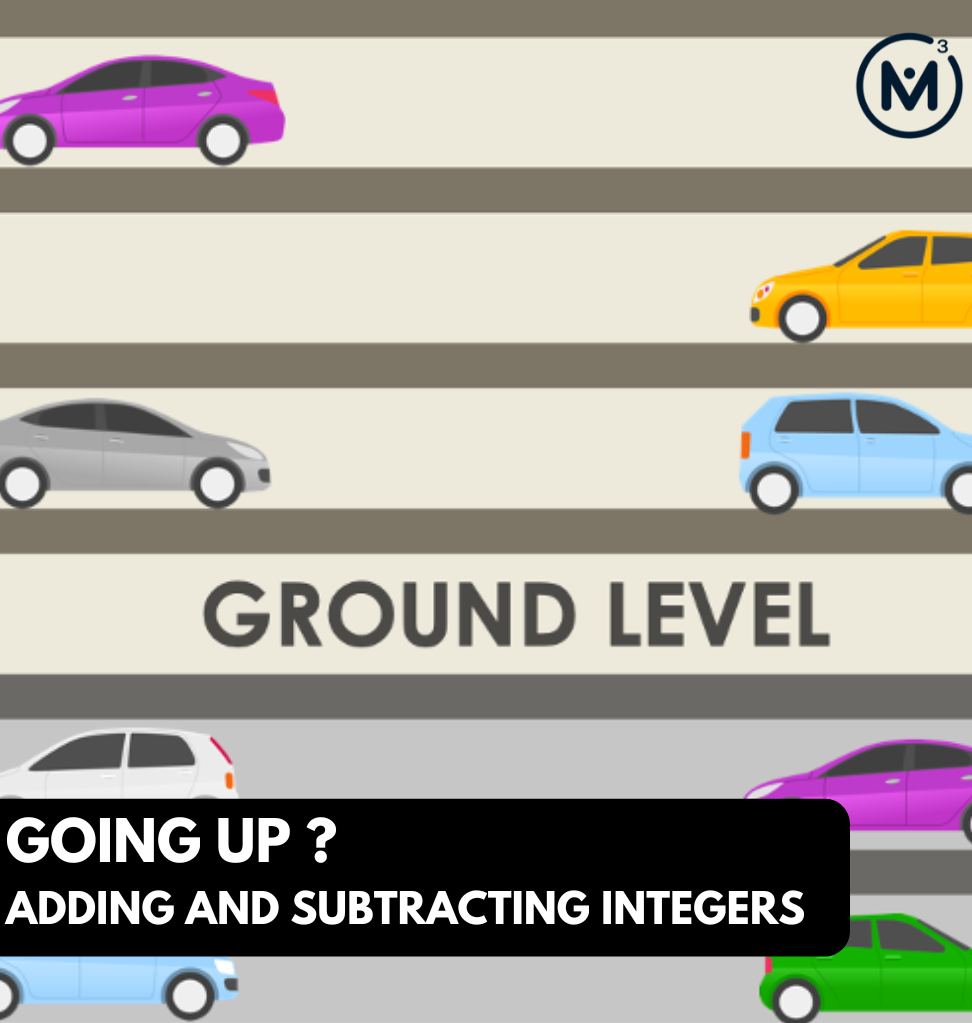### Access each lesson from this unit using the navigation links below

Through this math talk and purposeful practice, students are encouraged to use the number line to model the behaviour of adding and subtracting positive and negative integers. All the scenarios today follow the start, change and unknown result structure.

## Intentionality…

The purpose of the Day 2 activities is to reinforce key concepts from Day 1. Students will engage in a math talk and will have an opportunity to complete independent purposeful practice. The math talk and purposeful practice serve to develop a deeper understanding of the following big ideas.

• Situations involving addition and subtraction can be modelled using a number line;
• Integers include zero,  all whole numbers and their opposites;
• Integers represented on a number line show their relative distance from zero;
• Some addition and subtraction scenarios follow the structure of start, change and result;
• An integer can represent a change or a quantity;
• When a positive and a negative integer are added together, the result is positive if the absolute value of the positive integer is greater than the absolute value of the negative integer.

## Math Talk

In today’s math talk, present each parking garage scenario one at a time. In each scenario, encourage students to model the equation using a number line and solve. The purpose of today’s math talk is to make some generalizations about the result of various addition and subtraction scenarios involving positive and negative integers.

## Visual Math Talk Prompt #1

Show students the following visual math talk prompt and be prepared to pause the video where indicated:

This car is parked on level 5.

The car is moved down 6 levels.

What level is the car on now?

Write an equation to represent this scenario.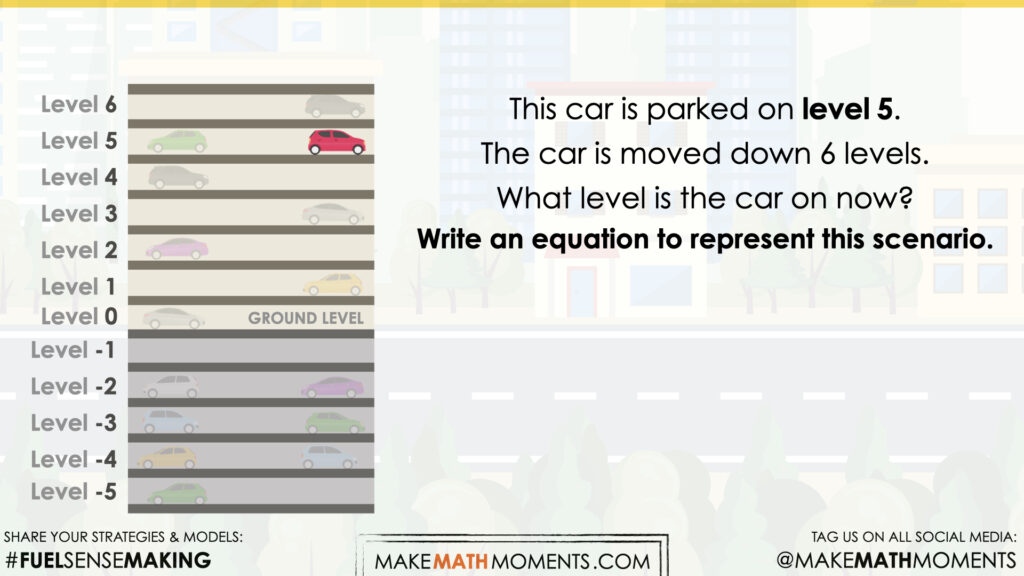In the first prompt from this string of related visual problems, students are asked to move the car down (or remove levels) from level 5 a total of 6 levels. The context was crafted so that it is clear that the car can move down below ground level and we’ll annotate those levels below ground using a negative sign.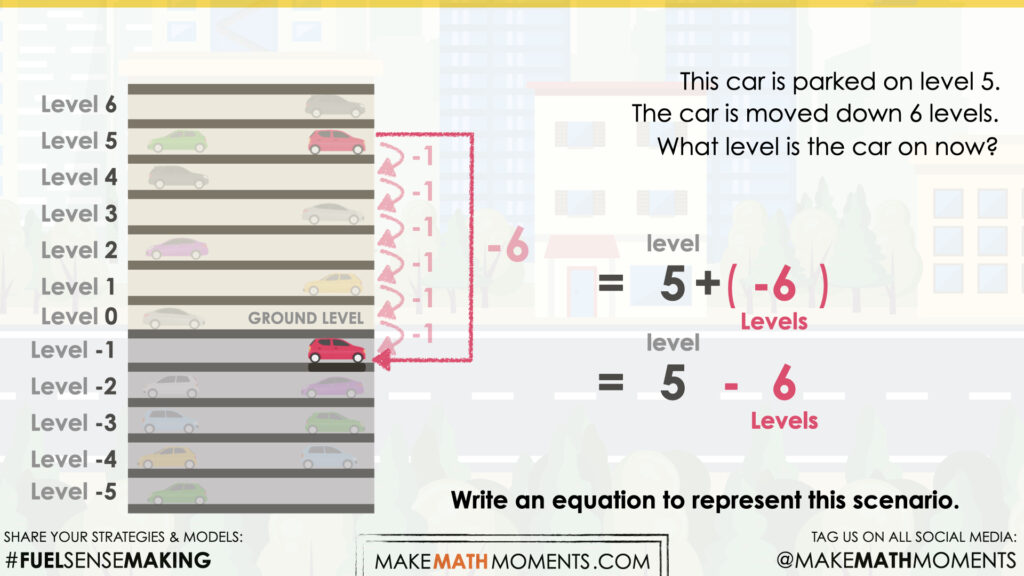When the car moves down 6 levels, we see there are only 5 levels until the ground floor (level 0) and one more level to go, bringing us to level -1.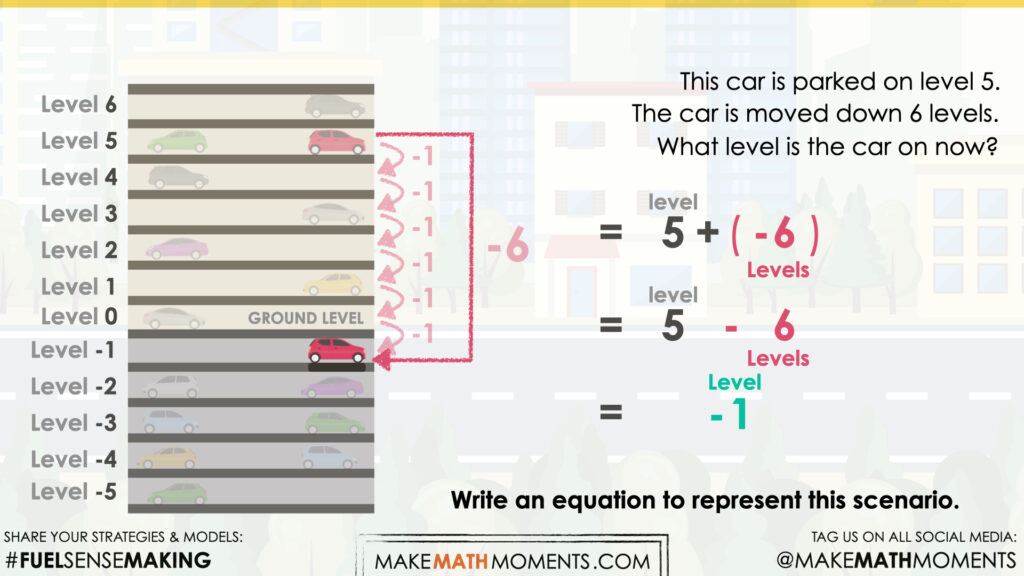Another approach is to leverage the zero principle by first decomposing -6 (or going down 6 levels) to -5 and -1 so level 5 and removing 5 levels cancel bringing the car to level 0. Then, we have a movement of -1 floors remaining to bring us to level -1.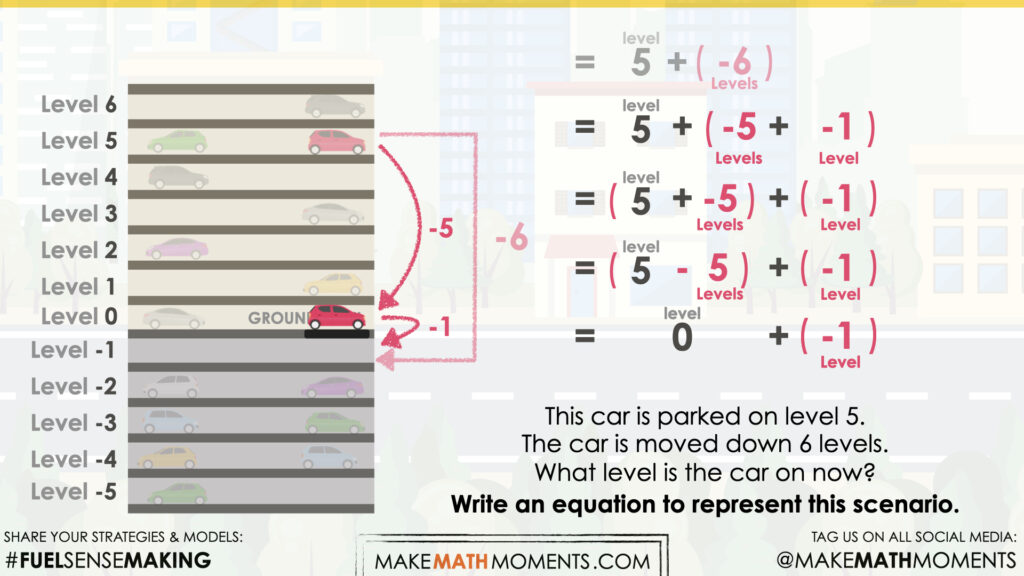Of course this action is very intuitive through the context of levels in a parking garage, however it is important to also annotate what we are doing using the symbolic representation for students to see the relationships involving integer operations of addition and subtraction.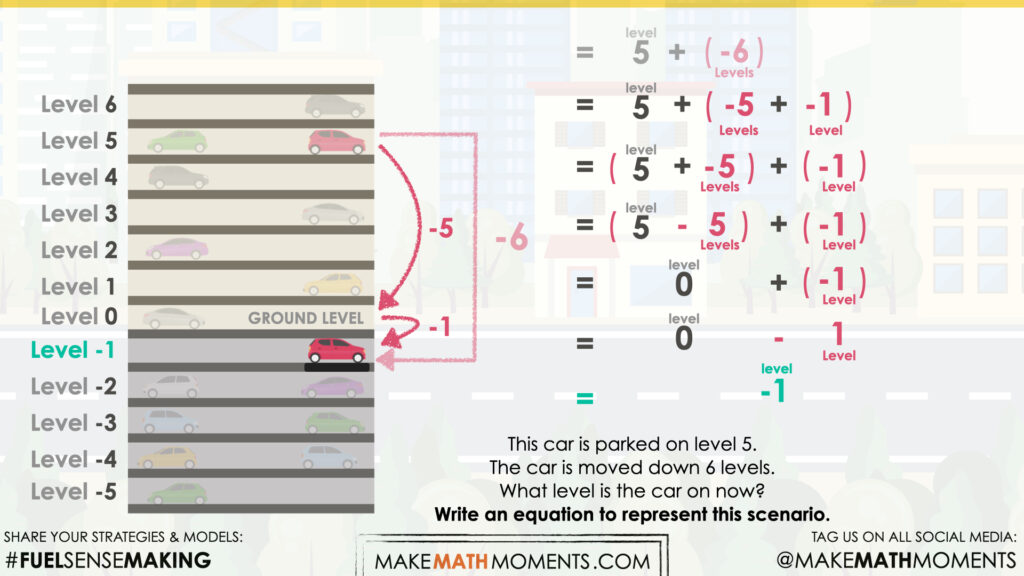Facilitator note:

The integers in this context can be interpreted as a change or as quantities of floors. For example, if the car starts on the fifth floor above ground level (5), and moves down 6 floors, this can be expressed as the addition of 6 negative floors [5 + (−6)], or a subtraction of 6 floors (5 − 6). Both statements result in the same answer (−1).

When a positive and a negative integer are added together, the result is negative if the absolute value of the positive integer is less than the absolute value of the negative integer.

## Visual Math Talk Prompt #2

Show students the following visual math talk prompt and be prepared to pause the video where indicated:

This car is parked on level -5.

The car is moved up 3 levels.

What level is the car on now?

Write an equation to represent this scenario.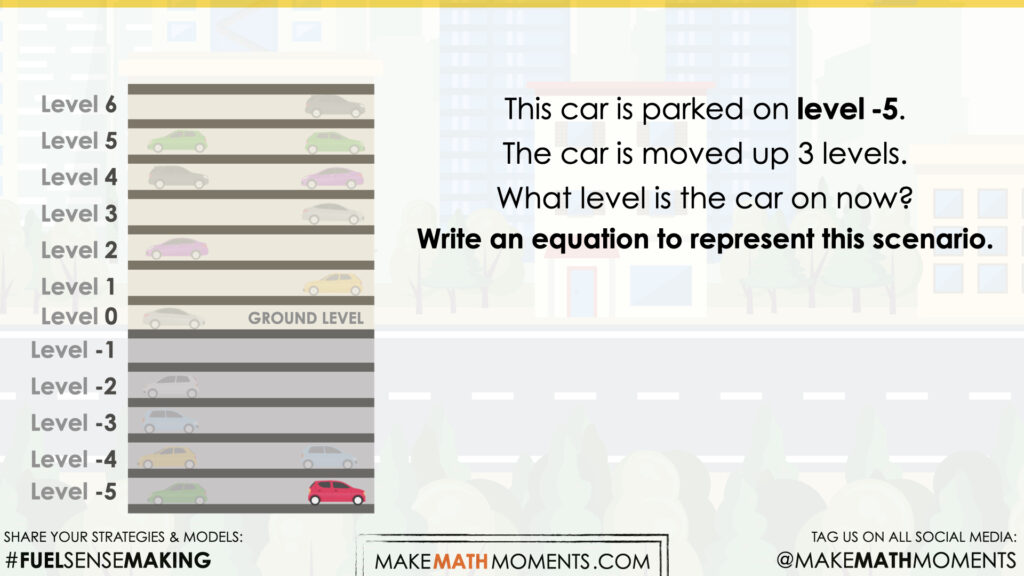Moving the car “up” 3 levels will bring the car closer to ground level. Worth noting is that -5 can be decomposed into -3 and -2, which can quickly allow us to reveal that the car should end up on level -2 as 3 + (-3) is equivalent to 0 due to the zero principle.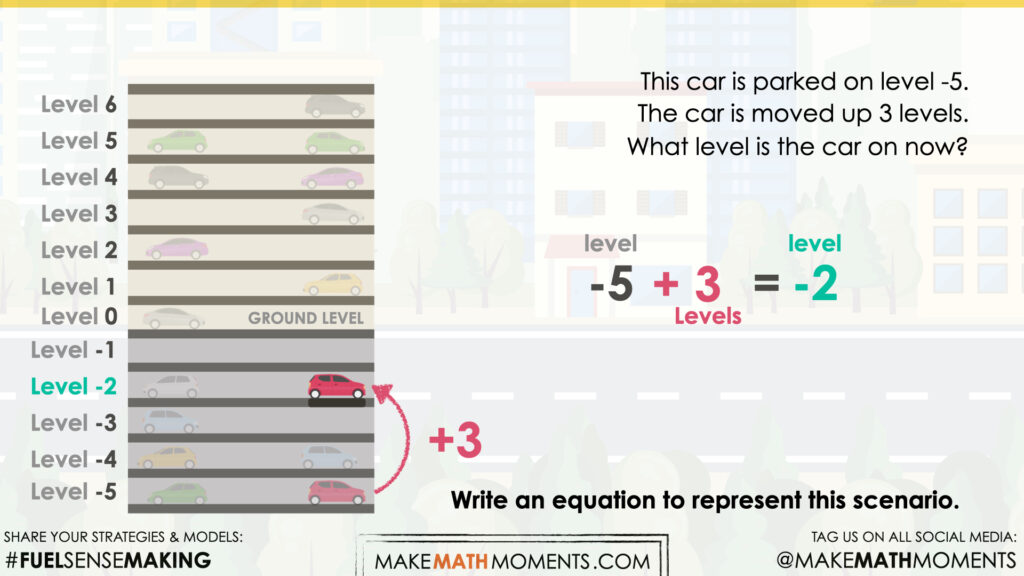Facilitator note:

In this scenario, we are adding a positive value to a negative integer. The absolute value of the positive is less than the absolute value of the negative. The result is negative.

## Visual Math Talk Prompt #3

Login/Join to access the entire Visual Prompt, downloadable slide decks and printable handouts for this lesson and all problem based units.

## While Students Are Practicing…

Login/Join to access the entire Teacher Guide, downloadable slide decks and printable handouts for this lesson and all problem based units.

## Question #1:

Login/Join to access the entire Teacher Guide, downloadable slide decks and printable handouts for this lesson and all problem based units.

## Question #2:

Login/Join to access the entire Teacher Guide, downloadable slide decks and printable handouts for this lesson and all problem based units.

## Question #3:

Login/Join to access the entire Teacher Guide, downloadable slide decks and printable handouts for this lesson and all problem based units.

## Question #4:

Login/Join to access the entire Teacher Guide, downloadable slide decks and printable handouts for this lesson and all problem based units.

## Question #5:

Login/Join to access the entire Teacher Guide, downloadable slide decks and printable handouts for this lesson and all problem based units.

Login/Join to access the entire Teacher Guide, downloadable slide decks and printable handouts for this lesson and all problem based units.

## Educator Discussion Area

Login/Join to access the entire Teacher Guide, downloadable slide decks and printable handouts for this lesson and all problem based units.

## Explore Our 60+ Problem Based Units

This Make Math Moments Lesson was designed to spark curiosity for a multi-day unit of study with built in purposeful practice, number talks and extensions to elicit and emerge strategies and mathematical models.

Dig into our other units of study and view by concept continuum, grade or topic!